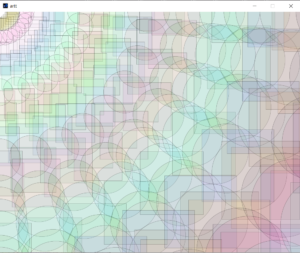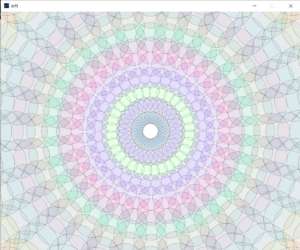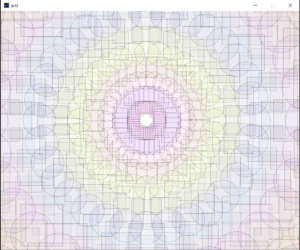# Simple Work of Art

#### Project Description:

Being creative on a deadline can be a struggle.

I did this design using both circles and “center” mode rects.

In the beginning, I tried using the normal formula for a circle to decide the midpoints of each layer of rect/circles, but for some reason, it did not work as required. So, after a long bugging session, I changed my equations, and I used sin and cos instead.

I started the code with the center circle starting at the top left corner, as it has (0,0) coordinates, making it easier to figure out what I was trying to do.After getting this part to work, I made the center point based on the center of the canvas.

I started with the centric shape being all circles with random-fill colors.But I then thought, why not make a random alternation between both squares and rectangles? It would make it more interesting.I also wanted to make it a little interactive, so I made the center point follow the mouse press as shown in the video.

And that is the end of my project’s story. 🙂

#### Code:

```  import java.lang.Math;

int dimWidth = 1000;
int dimHeight = 800;
int centerX = 500;
int centerY = 400;

void pattern(){
////x=0, y=0;

//println(x);
if(mousePressed){
centerX = mouseX;
centerY = mouseY;
}

for(int j=1; j<50 ; j++){
fill((int)random(0,255),(int)random(0,255),(int)random(0,255), 20);
int num =  (int)random(0,2);
strokeWeight(0.3);
for(int i=0;i<32;i++){
if (num==1){
circle(x_coordinate,y_coordinate,j*10+30);
}
else{
rectMode(CENTER);
rect(x_coordinate,y_coordinate, j*10+30, j*10+30);
}
}
}
}

void setup(){
size(1000,800);
background(255);
}

void draw(){
if (frameCount%10==0){
background(255);
pattern();
}
}```